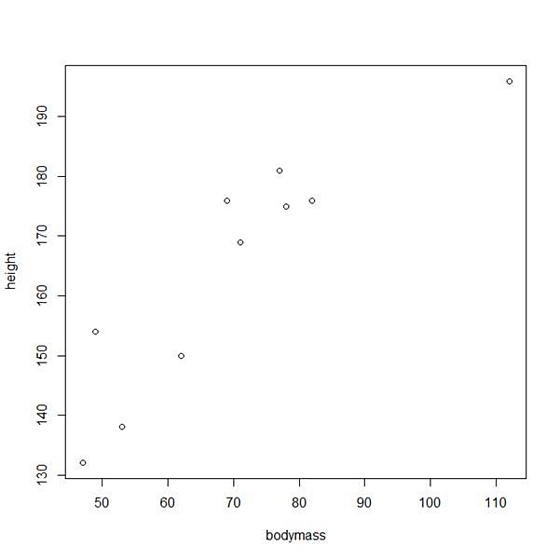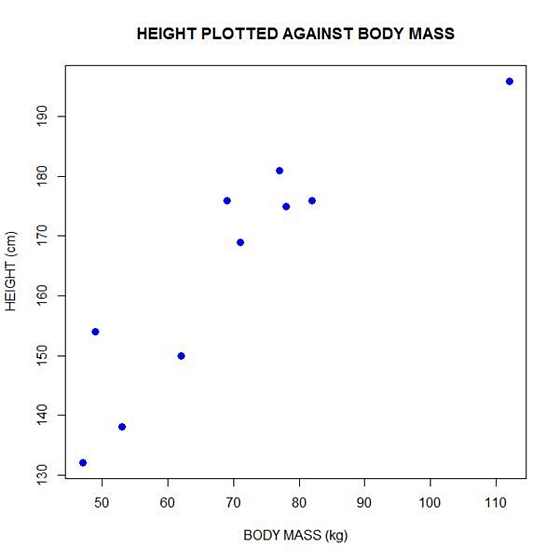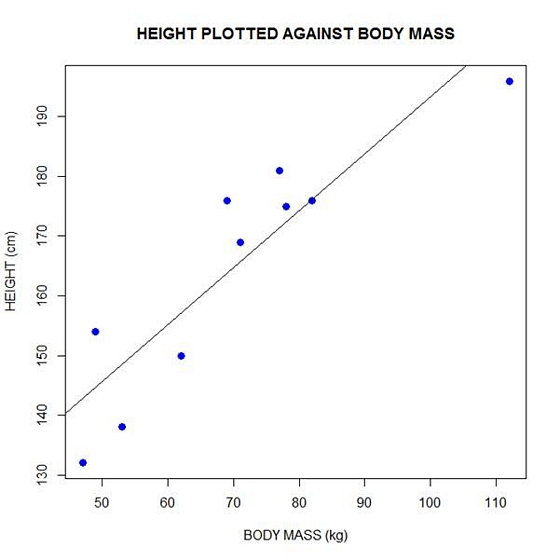# Linear Models in R: Plotting Regression LinesToday let’s re-create two variables and see how to plot them and include a regression line. We take height to be a variable that describes the heights (in cm) of ten people. Copy and paste the following code to the R command line to create this variable.

`height <- c(176, 154, 138, 196, 132, 176, 181, 169, 150, 175)`

Now let’s take bodymass to be a variable that describes the masses (in kg) of the same ten people. Copy and paste the following code to the R command line to create the bodymass variable.

`bodymass <- c(82, 49, 53, 112, 47, 69, 77, 71, 62, 78)`

Both variables are now stored in the R workspace. To view them, enter:

```height
 176 154 138 196 132 176 181 169 150 175```
```bodymass
 82 49 53 112 47 69 77 71 62 78```

We can now create a simple plot of the two variables as follows:

`plot(bodymass, height)`We can enhance this plot using various arguments within the plot() command. Copy and paste the following code into the R workspace:

```plot(bodymass, height, pch = 16, cex = 1.3, col = "blue", main = "HEIGHT PLOTTED AGAINST BODY MASS", xlab = "BODY MASS (kg)", ylab = "HEIGHT (cm)")

```In the above code, the syntax pch = 16 creates solid dots, while cex = 1.3 creates dots that are 1.3 times bigger than the default (where cex = 1). More about these commands later.

Now let’s perform a linear regression using lm() on the two variables by adding the following text at the command line:

```lm(height ~ bodymass)
Call:
lm(formula = height ~ bodymass)
Coefficients:
(Intercept)     bodymass
98.0054       0.9528```

We see that the intercept is 98.0054 and the slope is 0.9528. By the way – lm stands for “linear model”.

Finally, we can add a best fit line (regression line) to our plot by adding the following text at the command line:

`abline(98.0054, 0.9528)`

Another line of syntax that will plot the regression line is:

`abline(lm(height ~ bodymass))`In the next blog post, we will look at diagnosing our regression model in R.

David Lillis has taught R to many researchers and statisticians. His company, Sigma Statistics and Research Limited, provides both on-line instruction and face-to-face workshops on R, and coding services in R. David holds a doctorate in applied statistics.

1.rstudiohater says

hate r studio

2.Anjali Krishnan says

Hello,

I’m reaching out on behalf of the University of California – Irvine’s Office of Access and Inclusion. We are currently developing a project-based data science course for high school students. We would like your consent to direct our instructors to your article on plotting regression lines in R.

Thanks and best regards,
Anjali Krishnan

3.LUIZ AUGUSTO RODRIGUES says

I have an experiment to do de regression analisys, but i have some hibrids by many population. Then I have two categorical factors and one respost variable.
Could you help this case. If you have any routine or script this analisys and can share with me , i would be very grateful.

Luiz

4.Solomon says

Thanks a lot. this really helped.

5.Katarzyna says

Any idea how to plot the regression line from lm() results? I have more parameters than one x and thought it should be strightforward, but I cannot find the answer…

•theStig says

Seems you address a multiple regression problem (y = b1x1 + b2x2 + … + e). In this case, you obtain a regression-hyperplane rather than a regression line. For 2 predictors (x1 and x2) you could plot it, but not for more than 2.

6.Néstor Toledo says

Nice! Don’t you should log-transform the body mass in order to get a linear relationship instead of a power one?

7.rishvanth yokesh says

Bro, seriously it helped me a lot.
thank u yaar

Please note that, due to the large number of comments submitted, any questions on problems related to a personal study/project will not be answered. We suggest joining Statistically Speaking, where you have access to a private forum and more resources 24/7.